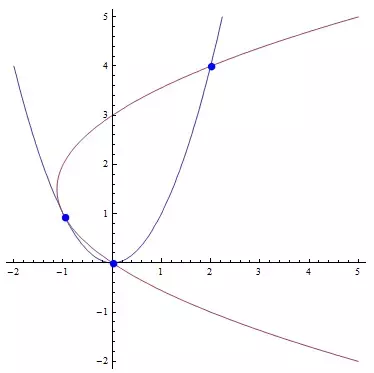# Creating a parabula from 3 points

What’s going on: I’m trying to make a jump that’s 6 units high, Delta Z can change and X doesn’t matter. I’ve looked at some tuturials, but can’t wrap my head around it. How do i make a parabula from 3 different points?

You want the player to jump in a parabola? What?

1 Like

I believe he wants to make a jump path in the shape of a parabola.

1 Like

What is a “jump path?” Generating a parabola to fit a shape isn’t too difficult but I’m not sure what is actually being made here.

Wouldn’t you just need two points? We already know the maximum height is 6 units.

one thing that makes it difficult is that’s it’s all programmed and cannot be done using roblox’s physics. not only do i not trust roblox’s physics, it’s also not apllicable for my project.
Also, i’ll need 3 points, because an infinite amount of parabulas can be drawn from 2 points

You can’t determine a parabola given three points because there are an infinite amount of them that can be drawn between three points. You’ll need more than just three random points.I personally think it would be much better to use kinematic equations for this.

2 Likes

This would work assuming you want to create a parabola to find the path of a jump from two given points and a given maximum height.

Let us assume that our original point be (x0, y0) and our final point be(x1, y1)

We’ll first need to find the vertex of the function.
The vertex will be of the form (xV, yV).
We can find the x-value of the vertex by finding the midpoint between the original and final point of the jump.

``````local xV = x1 - x0
``````

We know the y value of the vertex is 6, since it’s the height of the jump

``````local vY = 6
``````

Now that we have the zeros of the function and the vertex, we can create an equation using vertex form.

``````y = a(x - xV)^2 + yV
``````

We will plug in a point that is the length of the line from the original position to the final position to find the value of `a`.

``````dist = math.sqrt((x1 - x0)^2 + (y1 - y0)^2)
``````

We’ll plug this in as the x-value, and the y-value will be 0.

``````0 = a(dist - xV)^2 + xV
``````

Now we can simplify the equation and find the value for a, and then create an equation from there on.

3 Likes

i’ve already tried that, but this only gives me less control over the movement, wich would be kinda bad.

I’ll see if i can work from that explanation

I don’t really know how it would give you less control but, by less control I’m assuming the jump won’t really obey the laws of physics?

If so, yeah; you could also look into bezier curves which can be created from 3+ points.

Can’t you just change Player.Character.Humanoid.JumpPower?

Beziers aren’t efficient enough and would only be my last resort. and yes, it doesn’t really obey the laws of physics. it just needs to seem a believable enough jump for it to be what i need.

1 Like

I only wouldn’t even try scripting a jump through trying to script a parabola. It would be a very large amount of work just for a jump in my opinion, and depending on what type of game you are making and if it is a large one, it may cause lag every time someone jumps.

Instead you should just do it through velocity, and have the jump resemble a parabola instead of actually scripting a parabola path for the jump to follow.

Kinematics are going to give me very little control over the movement. I need full control of everywhere the player’s character goes. also, i’m not creating a game where the player can move in 3 directions and control their character directly in all 3 directions. only 1 can be controlled, one is constant and the other is for the jumping.

So you are making a platformer type game?

no, more a temple runner like game that i was going to complete in one day, wich i did. but i like the project so much it got out of hand, and that’s where this post comes from. i’m fitting it all into localscript and so fgar it’s working really well.